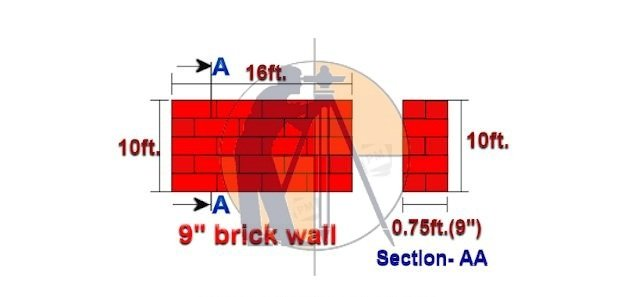Breaking News
Home / Civil Engineering / What will be the cost of 9″ brick wall masonry

# What will be the cost of 9″ brick wall masonry

## What will be the cost of 9″ brick wall masonry

Let us consider 9″ thick brick wall masonry as shown in the drawing for calculation purposes.Given data:

Length of the brick wall = 16ft.

Height of the brick wall = 10ft.

The thickness of the brick wall = 9″ = 0.75ft.

The total volume of the brick wall masonry

= length × height × thickness

= 16ft. × 10ft. × 0.75ft.

= 120 cu ft.

The total area of brick wall

= length × height

=16ft. × 10ft.

160 sq ft.

A.  Material calculation:

### Building Cinder Of Block Walls – Everything You Need to Know

before proceeding further.

No. of bricks required/ cu ft. of wall = 12.36 nos.

No. of Cement bags required/ cu ft of wall = 0.0353 bags

The volume of sand required/ cu ft. of wall = 0.2593 cu ft.

Note: The quantity of bricks, cement & sand per cu ft. is taken from the above-mentioned article.

1. No. of  bricks required

= [vol. of brick wall × bricks/ cu ft.]

=  [120 cu ft. × 12.36 nos.]

1483.20nos.

### Civil Engineering Measurements And Conversion Factors

2. No. of  cement bags required

= [vol. of brick wall × cement / cu ft.]

=  [120 cu ft. × 0.0353bags]

4.236 bags

3. The volume of sand required

= [vol. of brick wall × sand/ cu ft.]

=  [120 cu ft. × 0.2593 cu ft.]

31.116 cu ft.

## Other Post

3. ### How to Calculate the Cost of Paint for one Room

B.  Labor calculation:

The labor rate per sq ft. of the brick masonry ranges from INR 12/- to 20/- depending upon the region.

Let us consider an average rate of INR 16/– per sq ft.

The total cost of labor for 9″ brick masonry

= total area of brick masonry in sq ft.  × labor rate/ sq ft.

= 160 sq ft. × 16/-

INR 2,560/-

Now, let us calculate the total cost of brick masonry in table format.

 Sl. no. Item. Qty. Rate. in INR. Unit. Cost in INR. 1. Red brick 1483.20 8/- Nos. 11,865.60 2. Cement 4.236 380/- bag 1,609.68 3. Sand 31.116 60/- Cu ft. 1,866.96 4. Curing Lump-sum 250.00 5. Miscellaneous Lump-sum 200.00 6. The material cost = 15,792.24 7. Add 5% wastage = 789.61 8. Total material cost = 16,581.85 9. Labour cost = 2560.00 10. The total cost of 9″ brick masonry = 19,141.85

Note: To get accurate results, insert your regional market rate of materials & labor, in the above table.

The cost of double ( 9″ ) brick wall masonry/sq ft.

= [total brick masonry cost ÷ total brick wall area]

= 19,141.85 ÷ 160

= INR 119.64 /sq ft.

The cost of double ( 9″ ) brick wall masonry/cu ft.

= [total brick masonry cost ÷ total vol. of the brick wall]

= 19,141.85 ÷ 120

= INR 159.52 /cu ft.

2. ### How To Calculate The Self Weight Of Column & Beam

Thank you for going through this article❤. Have a good day .

If you have any quarries, you can ask me in the comment box .

## . Land Surveying & Architects

### THANKS.